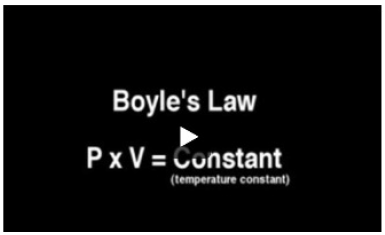# Problem: If a certain gas occupies a volume of 16 L when the applied pressure is 8.0 atm, find the pressure when the gas occupies a volume of 4.0 L.Express your answer to two significant figures and include the appropriate units.Watch the video that shows the relationship between pressure and volume for a particular gas. Robert Boyle was the first one to observe the change in the pressure of a gas when its volume is increased or decreased at a constant temperature and the change in the volume of a gas when its pressure is increased or decreased at a constant temperature.

###### FREE Expert Solution
91% (38 ratings)View Complete Written Solution
###### Problem Details

If a certain gas occupies a volume of 16 L when the applied pressure is 8.0 atm, find the pressure when the gas occupies a volume of 4.0 L.

Express your answer to two significant figures and include the appropriate units.

Watch the video that shows the relationship between pressure and volume for a particular gas. Robert Boyle was the first one to observe the change in the pressure of a gas when its volume is increased or decreased at a constant temperature and the change in the volume of a gas when its pressure is increased or decreased at a constant temperature.